# College Chemistry : Freezing and Melting

## Example Questions

### Example Question #1 : Freezing And Melting

Ice cubes are dropped into a glass of water. You notice that the glass of water becomes colder and condensation appears. Later in the day, you notice the glass of water is now room temperature and there is no more condensation. Which of the following concepts describes this process?

Thermal equilibrium: Thermal energy of the system is equal to thermal energy of the surroundings.

Work: the system did work on the surroundings and lost heat, resulting in a colder glass of water with condensation

Energy: potential energy of the ice was converted to kinetic energy of the water.

Chemical Potential Energy: intermolecular forces of the water broke apart the intramolecular forces within the ice and absorbed the excess energy.

Thermal equilibrium: specific heat capacity of ice is greater than water and the ice melts quickly.

Thermal equilibrium: Thermal energy of the system is equal to thermal energy of the surroundings.

Explanation:

Thermal equilibrium: Thermal energy of the system is equal to thermal energy of the surroundings. Heat is defined as the energy transfer resulting from differences in thermal energy. Heat always flows from higher temperature to lower temperature. Heat transfer between a system and its surroundings stop when they reach thermal equilibrium, or when there is no difference in thermal energy. In this case, ice was dropped into the cup. Initially, the ice increases the temperature of the water, creating a cold glass with condensation. However, energy from the surroundings flow into the system (the glass of water) due to thermal difference and warm the glass of water until the two reach thermal equilibrium. At thermal equilibrium, there is no ice, no condensation, and the water temperature is room temperature.

### Example Question #2 : Freezing And Melting

Suppose that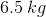of a certain compound is needed in order to lower the freezing point of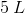of water by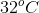. What is the molar mass of this compound?

Note: Liquid water has a density of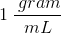and a molal freezing-point depression constant of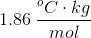.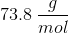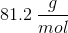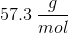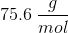Explanation:

In this question, we're told that a certain amount of a compound dissolved in water lowers the water's freezing point. We're asked to determine the molar mass of the compound.

First, we must recognize this as a freezing point depression problem. Recall that freezing point depression is one of the colligative properties associated with dissolving solute into a solvent such as water.

To begin, we need to use the equation for freezing point depression, which states that the change in freezing point is proportional to both the molality of the solution as well as the molal freezing point depression constant for the solvent in question (in this case, water).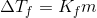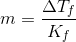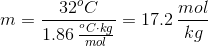The answer we have just calculated above is the molality, which is a different way of expressing concentration than molarity. Molality is expressed as the number of moles of solute per kilogram of solvent, whereas molarity is the number of moles of solute divided by the total volume of the solution.

The next thing we need to do is find out how many total moles of solute are present in the solvent water. To do this, we need to use the volume of water provided to us in the question, along with the density of water, to calculate the mass of water present. Together with the molality calculated above, this will allow us to know the total number of moles in solution.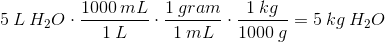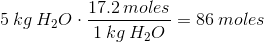Now that we know the total number of moles of solute (our compound) that exists in solution, we can use that information, together with the total mass of the compound given to us in the question stem, to calculate the compound's molar mass.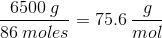### Example Question #3 : Freezing And Melting

Ice can be used to counter the effects of overeating. How many kilograms of ice at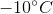would one have to eat in order to cancel the effect of eating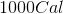? Assume body temperature to be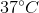.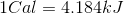Specific heat of water =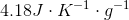Specific heat of ice =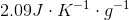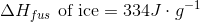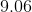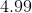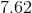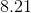Explanation:

Recall that the heat gained by ice must be equal to the heat from the food.

Start by calculating the heat from the food by converting the Calories into joules.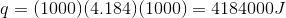Next, calculate the heat gained by the ice. Take this in three steps:

1. Heating the ice fromto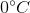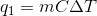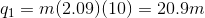2. Ice melting.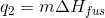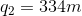3. Raising the temperature of water fromto.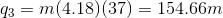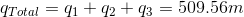Set this value equal to the heat from the food and solve for the mass.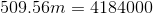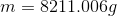Convert to kilograms.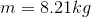### Example Question #4 : Freezing And Melting

As heat energy is added to a cube of ice, it begins to melt into liquid water. Which of the following correctly identifies the change in temperature and the change in internal energy of the ice as heat is added to it?

The temperature increases while the internal energy remains constant

The temperature and the internal energy both increase

The temperature and the internal energy both remain constant

The temperature remains constant while the internal energy increases